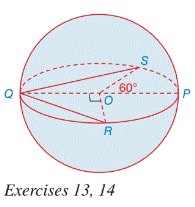Chapter 9.4, Problem 13E### Elementary Geometry for College St...

6th Edition
Daniel C. Alexander + 1 other
ISBN: 9781285195698

#### Solutions

Chapter
Section### Elementary Geometry for College St...

6th Edition
Daniel C. Alexander + 1 other
ISBN: 9781285195698
Textbook Problem
3 views

# In sphere O, the length of radius O P - is 6 in. Find the length of the chord:a) Q R - if ∠ Q O R = 90 ° b) Q S - if ∠ S O P = 60 °To determine

a)

To find:

The length of the chord.

Explanation

Approach:

A sphere is a three dimensional solid figure, which is made up of all the points in space, which lie at a common distance, called the radius, from a fixed point called the center of the sphere.

The surface area of a sphere S =4πr2, where r is the radius of the sphere.

All solid bodies occupy space. The measure of occupied space is called the volume of the object.

Volume of a sphere V = 43πr3.

The Pythagorean theorem states that in a right angled triangle, the square of the hypotenuse is equal to the sum of the squares of the other two sides. The longest side of the right angle triangle is called the hypotenuse.

Calculation:

In sphere O, the length of radius OP=6 in.

In QOR,m.QOR is a right angled triangle.

QO = QR = radius = 6 in.

QR is the hypotenuse.

Using the Pythagorean theorem, side2+side2=

To determine

b)

To find:

The length of the chord.

### Still sussing out bartleby?

Check out a sample textbook solution.

See a sample solution

#### The Solution to Your Study Problems

Bartleby provides explanations to thousands of textbook problems written by our experts, many with advanced degrees!

Get Started

#### Find more solutions based on key concepts